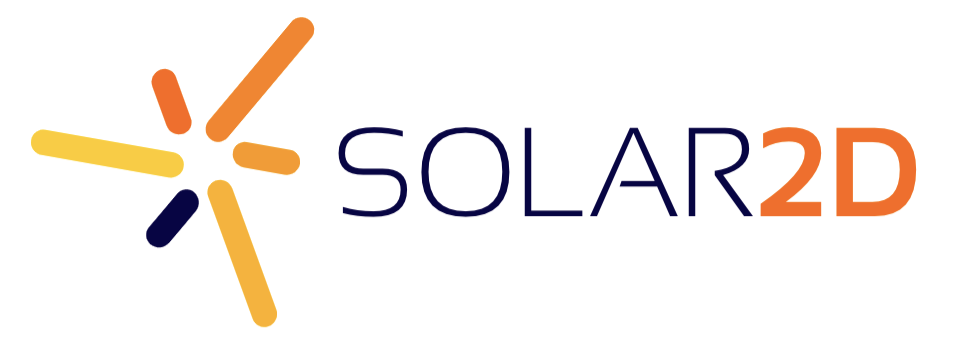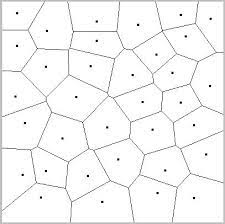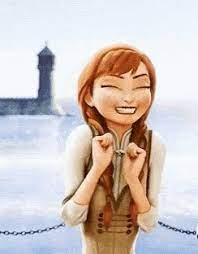# Duplicate display object

Hello.

In a screen transition, I would like to split the screen into several parts and move each part in different ways.

I will take a screenshot (capture) and then use masks.

Do I need as many screenshots as I have screen parts and if so, how do I duplicate the capture?
Or is it possible to draw a polygon and fill it with a texture I could rotate ?

Thank’s

You could try putting the capture into a canvas.

If your parts will be rectangular, you could just make a sprite sheet, create each “frame”, and go from there.

Otherwise, say with a polygon, you’re bound to get a little weirdness since the image will center and resize according to the polygon’s bounding box.

If you didn’t need the vertex userdata for something else, you might do a shader like so:

``````local kernel = { category = "filter", group = "shard", name = "basic_uv" }

kernel.vertexData = {
{
name = "min_u",
default = 0,
min = 0,
max = 1,
index = 0
},

{
name = "min_v",
default = 0,
min = 0,
max = 1,
index = 1
},

{
name = "max_u",
default = 1,
min = 0,
max = 1,
index = 2
},

{
name = "max_v",
default = 1,
min = 0,
max = 1,
index = 3
},
}

kernel.vertex = [[
P_POSITION vec2 VertexKernel (P_POSITION vec2 position)
{
v_TexCoord = mix(CoronaVertexUserData.xy, CoronaVertexUserData.zw, CoronaTexCoord);

return position;
}
]]

graphics.defineEffect(kernel)

local r1 = display.newImageRect("Front3.png", 250, 250) -- use canvas.filename, canvas.baseDir
local r2 = display.newImageRect("Front3.png", 250, 250) -- ditto

r1.x, r1.y = 200, 200
r2.x, r2.y = 200, 700

r1.fill.effect = "filter.shard.basic_uv"
r2.fill.effect = "filter.shard.basic_uv"

r1.fill.effect.min_u = .6 -- start 60% to the right in the image

r2.fill.effect.min_v = .3 -- start 30% of the way down...
r2.fill.effect.max_v = .8 -- ...and finish 80%
``````

With rect distortion, you could probably get the polygonal behavior you need. There are other possible approaches too, but they get a little weird.Hum.

In fact, I’d like de split the screen with something like voronoy graphthen give each polygon a direction and a rotation!
I think it is not possible to rotate the texture!

I think the mask is the best option if I succeed to dynamically generate a mask bitmapYes canvas seem’s to the good way to do do what I want !
Finally no because setMask cannot take anything else than an image…

Thank’s

I’ll be to be able to do what I want just With fill if…

if it’s possible to give to fill a snapshoot

``````local polygon = {0, 0, 106.4, 0, 109.57, 89.35, 77.33, 110.33, 0, 89.21}

local paint = {
type = "image",
filename = "world2.jpg"
}

local p2 = display.newPolygon(0, 0, polygon)
p2.x = p2.width * 0.5
p2.y = p2.height * 0.5
p2.fill = paint
p2.fill.x = -0.5 + ((p2.width / 960) * 0.5)
p2.fill.y = -0.5 + ((p2.height / 960) * 0.5)
p2.fill.scaleX = 960 / p2.width
p2.fill.scaleY = 640 / p2.height
p2.rotation = 20
``````

That’s perfect, but I need to not use an image but a dynamic snapshoot as fill pattern !

Do you think it’s possible ?

Yes``````local tex = graphics.newTexture{ type = "canvas", width = cntWidth, height = cntHeight }
local img = display.newImage('world2.jpg')
tex:draw(img)
tex:invalidate()

local paint = {
type = "image",
filename = tex.filename,
baseDir = tex.baseDir
}

local p2 = display.newPolygon(0, 0, polygon)
p2.x = p2.width * 0.5
p2.y = p2.height * 0.5
p2.fill = paint
p2.fill.x = -0.5 + ((p2.width / 960) * 0.5)
p2.fill.y = -0.5 + ((p2.height / 960) * 0.5)
p2.fill.scaleX = 960 / p2.width
p2.fill.scaleY = 640 / p2.height
p2.rotation = 20
``````

That’s possible !Here is the final result !

1 Like

Here is the source code !

1 Like

This topic was automatically closed 180 days after the last reply. New replies are no longer allowed.